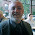## Friday, September 23, 2016

### Building Supply and Demand Graphs in Python

When I first started teaching, I grew frustrated with the process of creating visualizations. Microsoft office graphs look tacky. I wanted something that is can be standardized for making graphs of supply and demand. I also wanted it to be customizable and not be corrupted by the name of the company that provided the means for creating the graph (see here). After having used Python and the matplot library to make other visualizations, I realized that I could use similar code for making a generic supply and demand graph. You can customize Matplotlib's graphs to a significant degree.

If you are afraid of programming, never fear. This is a great place to start. Just download Anaconda and use this template. The code below is filled with notes to help you fill out the template. The final graph is depicted above:
 ``` 1 2 3 4 5 6 7 8 9 10 11 12 13 14 15 16 17 18 19 20 21 22 23 24 25 26 27 28 29 30 31 32 33 34 35 36 37 38 39 40 41 42 43 44 45 46 47 48 49 50 51 52 53 54 55 56 57 58 59 60 61 62 63 64 65 66 67 68 69 70 71 72 73 74 75 76 77 78 79 80 81 82 83 84 85 86 87 88 89 90 91 92 93 94 95 96 97 98 99 100 101 102 103``` ```from __future__ import print_function import numpy as np import matplotlib.pyplot as plt from matplotlib.backends.backend_pdf import PdfPages pp = PdfPages('LaborSupplyAndDemandFloor.pdf') # "supply", "demand", "floor" and "equilibrium" are all numpy arrays # created at the bottom of the script. # the supplyAndDemandFloor method is also called at the bottom of the script def supplyAndDemandFloor(supply, demand, floor, equilibrium): fig = plt.figure(dpi=128, figsize=(10,6)) # Plot supply and demand curves # Must instantiate numpy arrays -- see lines 85 and 87 plt.plot(supply, 'k-', linewidth=3) plt.plot(demand, 'k-', linewidth=3) # The length of the equilibrium line must match the horizontal coordinate # of the equilibrium quanity -- see lines 98, 100 plt.plot(equilibrium, 'k--', linewidth=1.5) # The floor is a horizontal line -- see lines 90, 94 plt.plot(floor, 'r--',label= "Price Floor", linewidth=1.5) # plt plt takes the form like: # plt.plot((x1,x2)),(y1,y2), 'r-', linewidth = x) # e.g., for command on line ##: # create a vertical line at x1 = 2000, y2 = 2000, y1 = 0, y2 = 8000 plt.plot((2000, 2000), (0, 8000), 'r--', linewidth=1.5) plt.plot((8000, 8000), (0, 8000), 'r--', linewidth=1.5) plt.plot((5000, 5000), (0, 5000), 'k--', linewidth=1.5) # frame = plt.gca() required to use commands for removing # values on axes frame = plt.gca() # remove axis values frame.axes.get_xaxis().set_visible(False) frame.axes.get_yaxis().set_visible(False) plt.title('Pizza Market', fontsize = 32) # p variable on top of y-axis plt.text(-500, 10000, "\$p\$", fontsize=24) # surplus price variable plt.text(-550, 7900, "\$p_s\$", fontsize=24) # equilibrium price variable plt.text(-550, 5000, "\$p_e\$", fontsize=24) # identify supply curve plt.text(8200, 9000,"\$S_p\$", fontsize = 24) # identify demand curve plt.text(8200, 2000,"\$D_p\$", fontsize = 24) # quantity demanded at surplu price plt.text(1800, -650, "\$Q_d\$", fontsize=24) # quantity supplied at surplus price plt.text(7800, -650, "\$Q_s\$", fontsize=24) # Qs = Qd at equilibrium price plt.text(4800, -650, "\$Q_e\$", fontsize=24) # mark that Q is represented on horizontal axis plt.text(10000, -650, "\$Q\$", fontsize=24) # label the axes plt.xlabel("Pizza", fontsize=20) plt.ylabel("Price", fontsize = 20) plt.tick_params(axis='both', which='major', labelsize=16) # plt.legend(floor, loc='upper right') # save PDF of figure # you can also save to image file # pdf is useful if multiple graphs are created pp.savefig(fig) # close PDF pp.close() # Instantiate array with supply curve points supply = np.arange(10000) # ... demand curve points demand = np.arange(10000,0, -1) # ... for price floor line priceFloor = np.arange(1, 10000) # set value of all points value of price floor # value of prices are arbitrary since we dropped # axis values priceFloor[priceFloor > 0] = 8000 # ... for equilibrium price, length of this array # is equal to the equilibrium quantity priceEquilibrium = np.arange(1, 5000) # set all values to the equilibrium price priceEquilibrium[priceEquilibrium > 0] = 5000 # finally, call method to produce the graph supplyAndDemandFloor(supply,demand, priceFloor, priceEquilibrium) ```

#### 1 comment:

1.Too many lines of code! You should figure out intermediate function to cut out 90% of them. I'll talk about this with you if you would like.